## December 22, 2015

#### Posted by John BaezA few years ago, after hearing Susan Holmes speak about the mathematics of phylogenetic trees, I became interested in their connection to algebraic topology. I wrote an article about it:

In trying to the make the ideas precise I recruited the help of Nina Otter, who was then a graduate student at ETH Zürich. She came to Riverside and we started to work together.

Now Nina Otter is a grad student at Oxford working on mathematical biology with Heather Harrington. I visited her last summer and we made more progress… but then she realized that our paper needed another big theorem, a result relating our topology on the space of phylogenetic trees to the topology described by Susan Holmes and her coauthors here:

It took another half year to finish things up. I could never have done this myself.

But now we’re done! Here’s our paper:

Let me tell you the basic idea….

Trees are important, not only in mathematics, but also biology. The most important is the ‘tree of life’ relating all organisms that have ever lived on Earth. Darwin drew this sketch of it in 1837: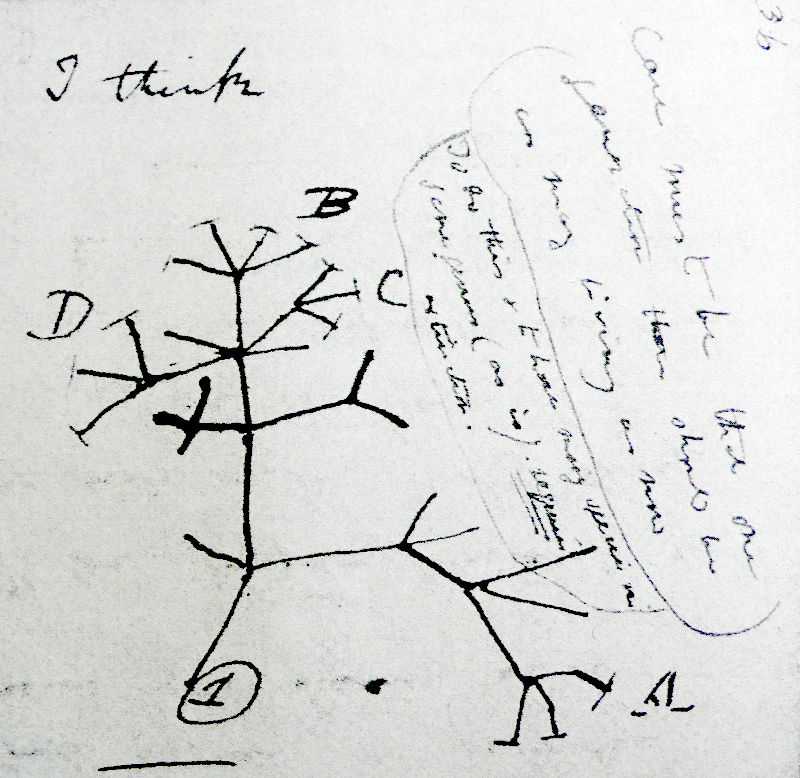He wrote about it in On the Origin of Species:

The affinities of all the beings of the same class have sometimes been represented by a great tree. I believe this simile largely speaks the truth. The green and budding twigs may represent existing species; and those produced during former years may represent the long succession of extinct species. At each period of growth all the growing twigs have tried to branch out on all sides, and to overtop and kill the surrounding twigs and branches, in the same manner as species and groups of species have at all times overmastered other species in the great battle for life.

Now we know that the tree of life is not really a tree in the mathematical sense. One reason is ‘endosymbiosis’: the incorporation of one organism together with its genetic material into another, as probably happened with the mitochondria in our cells and also the plastids that hold chlorophyll in plants. Another is ‘horizontal gene transfer’: the passing of genetic material from one organism to another, which happens frequently with bacteria. So, the tree of life is really a thicket: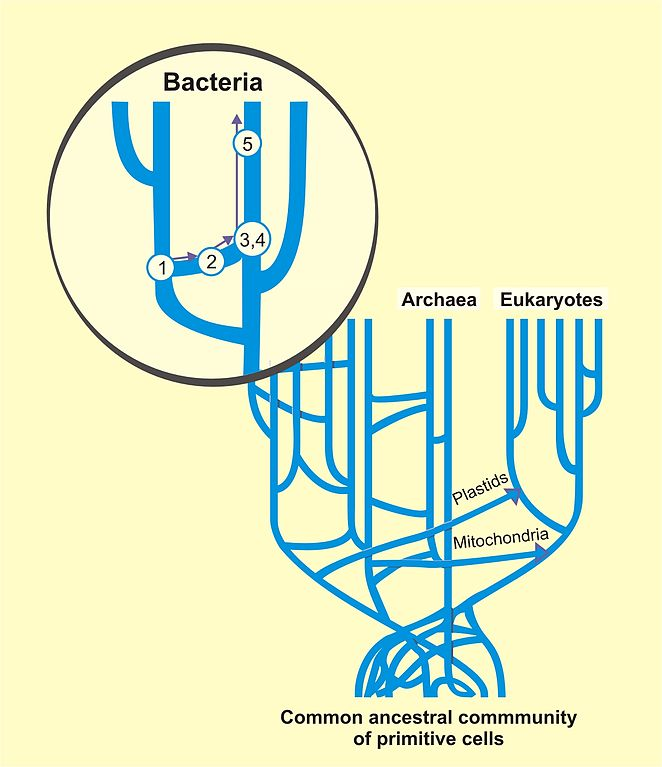But a tree is often a good approximation, especially for animals and plants in the last few hundred million years. Biologists who try to infer phylogenetic trees from present-day genetic data often use simple models where:

• the genotype of each species follows a random walk, but
• species branch in two at various times.

These are called ‘Markov models’. The simplest Markov model for DNA evolution is the Jukes–Cantor model. Consider one or more pieces of DNA having a total of $n$ base pairs. We can think of this as a string of letters chosen from the set {A,T,C,G}:

…ATCGATTGAGCTCTAGCG…

As time passes, the Jukes–Cantor model says the DNA changes randomly, with each base pair having the same constant rate of randomly flipping to any other. So, we get a Markov process on the set

$X = \{ A,T,C,G \}{}^N$

However, a species can also split in two. So, given current-day genetic data from various species, biologists try to infer the most probable tree where, starting from a common ancestor, the DNA in question undergoes a random walk most of the time but branches in two at certain times.

To formalize this, we can define a concept of ‘phylogenetic tree’. Our work is based on the definition of Billera, Holmes and Vogtmann, though we use a slightly different definition, for reasons that will soon become clear. For us, a phylogenetic tree is a rooted tree with leaves labelled by numbers $1,2, \dots, n$ and edges labelled by ‘times’ or, geometrically speaking, ‘lengths’ in $[0, \infty)$. We require that:

• the length of every edge is positive, except perhaps for ‘external edges’: that is, edges incident to the leaves or root;
• there are no 1-ary vertices.

For example, here is a phylogenetic tree with 5 leaves: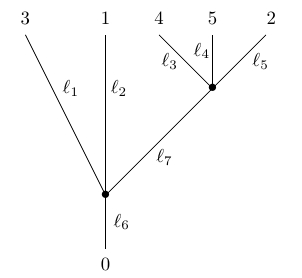where $\ell_1, \dots, \ell_6 \ge 0$ but we demand that $\ell_7 > 0$. We draw the vertices as dots. We do not count the leaves and the root as vertices, and we label the root with the number $0$. We cannot collapse edges of length zero that end at leaves, since doing so would eliminate those leaves. Also note that the embedding of the tree in the plane is irrelevant, so this counts as the same phylogenetic tree: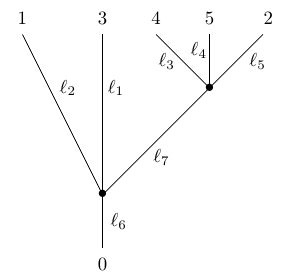In applications to biology, we are often interested in trees where the total distance from the root to the leaf is the same for every leaf, since all species have evolved for the same time from their common ancestor. These are mathematically interesting as well, because then the distance between any two leaves defines an ultrametric on the set of leaves. However, more general phylogenetic trees are also interesting—and they become essential when we construct an operad whose operations are phylogenetic trees.

Let ${Phyl}_n$ be the set of phylogenetic trees with $n$ leaves. This has a natural topology, which we explain in Section 6 of our paper. For example, here is a continuous path in ${Phyl}_4$ where we only change the length of one internal edge, reducing it until it becomes zero and we can collapse it: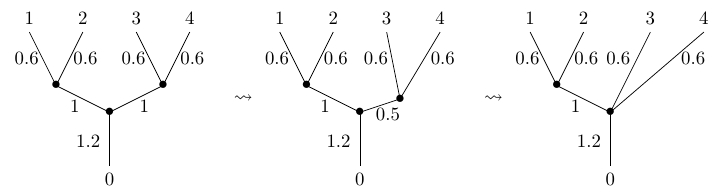Phylogenetic trees reconstructed by biologists are typically binary. When a phylogenetic tree appears to have higher arity, sometimes we merely lack sufficient data to resolve a higher-arity branching into a number of binary ones. With the topology we are using on ${Phyl}_n$, binary trees form an open dense set of ${Phyl}_n$, except for ${Phyl}_1$. However, trees of higher arity are still important, because paths, paths of paths, etc. in ${Phyl}_n$ are often forced to pass through trees of higher arity.

Billera, Holmes and Vogtmann focused their attention on the set $\mathcal{T}_n$ of phylogenetic trees where lengths of the external edges—edges incident to the root and leaves—are fixed to a constant value. They endow $\mathcal{T}_n$ with a metric, which induces a topology on $\mathcal{T}_n$, and there is a homeomorphism

${Phyl}_n \cong \mathcal{T}_n \times [0,\infty)^{n+1}$

where the data in $[0,\infty)^{n+1}$ describe the lengths of the external edges in a general phylogenetic tree.

In algebraic topology, trees are often used to describe the composition of $n$-ary operations. This is formalized in the theory of operads. An ‘operad’ is an algebraic stucture where for each natural number $n=0,1,2,\dots$ we have a set $O_n$ whose elements are considered as abstract $n$-ary operations, not necessarily operating on anything yet. An element $f \in O_n$ can be depicted as a planar tree with one vertex and $n$ labelled leaves: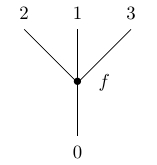We can compose these operations in a tree-like way to get new operations: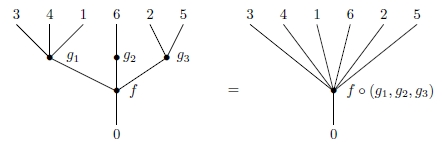and an associative law holds, making this sort of composite unambiguous:There are various kinds of operads, but in our paper the operads are always ‘unital’, having an operation $1 \in O_1$ that acts as an identity for composition. They are also ‘symmetric’, meaning there is an action of the symmetric group $S_n$ on each set $O_n$, compatible with composition. Further, they are ‘topological’, meaning that each set $O_n$ is a topological space, with composition and permutations acting as continuous maps.

In Section 5 we prove that there is an operad ${Phyl}$, the ‘phylogenetic operad’, whose space of $n$-ary operations is ${Phyl}_n$. This raises a number of questions:

• What is the mathematical nature of this operad?
• How is it related to ‘Markov processes with branching’?
• How is it related to known operads in topology?

Briefly, the answer is that ${Phyl}$ is the coproduct of ${Com}$, the operad for commutative topological semigroups, and $[0,\infty)$, the operad having only unary operations, one for each $t \in [0,\infty)$. The first describes branching, the second describes Markov processes. Moreover, ${Phyl}$ is closely related to the Boardmann–Vogt $W$ construction applied to ${Com}$. This is a construction that Boardmann and Vogt applied to another operad in order to obtain an operad whose algebras are loop spaces.

To understand all this in more detail, first recall that the raison d’être of operads is to have ‘algebras’. The most traditional sort of algebra of an operad $O$ is a topological space $X$ on which each operation $f \in O_n$ acts as a continuous map

$\alpha(f)\colon X^n \to X$

obeying some conditions: composition, the identity, and the permutation group actions are preserved, and $\alpha(f)$ depends continuously on $f$. The idea is that the abstract operations in $O$ are realized as actual operations on the space $X$.

In this paper we instead need algebras of a linear sort. Such an algebra of $O$ is a finite-dimensional real vector space $V$ on which each operation $f \in O_n$ acts as a multilinear map

$\alpha(f)\colon V^n \to X$

obeying the same list of conditions. We can also think of $\alpha(f)$ as a linear map

$\alpha(f)\colon V^{\otimes n} \to V$

where $V^{\otimes n}$ is the $n$th tensor power of $V$.

We also need ‘coalgebras’ of operads. The point is that while ordinarily one thinks of an operation $f \in O_n$ as having $n$ inputs and one output, a phylogenetic tree is better thought of as having one input and $n$ outputs. A coalgebra of $O$ is a finite-dimensional real vector space $V$ on which operation $f \in O_n$ gives a linear map

$\alpha(f) \colon V \to V^{\otimes n}$

obeying the same conditions as an algebra, but ‘turned around’.

The main point of this paper is that the phylogenetic operad has interesting coalgebras, which correspond to how phylogenetic trees are actually used to describe branching Markov processes in biology. But to understand this, we need to start by looking at coalgebras of two operads from which the phylogenetic operad is built.

By abuse of notation, we will use $[0,\infty)$ as the name for the operad having only unary operations, one for each $t \in [0,\infty)$, with composition of operations given by addition. A coalgebra of $[0,\infty)$ is a finite-dimensional real vector space $V$ together with for each $t \in [0,\infty)$ a linear map

$\alpha(t) \colon V \to V$

such that:

• $\alpha(s+t) = \alpha(s) \alpha(t)$ for all $s,t \in [0,\infty)$
• $\alpha(0) = 1_V$
• $\alpha(t)$ depends continuously on $t$.

Analysts usually call such a thing a ‘continuous one-parameter semigroup’ of operators on $V$.

Given a finite set $X$, a ‘Markov process’ or ‘continuous-time Markov chain’ on $X$ is a continuous one-parameter semigroup of operators on $\mathbb{R}^X$ such that if $f \in \mathbb{R}^X$ is a probability distribution on $X$, so is $\alpha(t) f$ for all $t \in [0,\infty)$. Equivalently, if we think of $\alpha(t)$ as a $X \times X$ matrix of real numbers, we demand that its entries be nonnegative and each column sum to 1. Such a matrix is called ‘stochastic’. If $X$ is a set of possible sequences of base pairs, a Markov process on $X$ describes the random changes of DNA with the passage of time. We shall see that any Markov process on $X$ makes $\mathbb{R}^X$ into a coalgebra of $[0,\infty)$.

This handles the Markov process aspect of DNA evolution; what about the branching? For this we use ${Com}$, the unique operad with one $n$-ary operation for each $n$ > 0. Algebras of ${Com}$ are not-necessarily-unital commutative algebras: there is only one way to multiply $n$ elements for $n$ > 0.

For us what matters most is that coalgebras of ${Com}$ are finite-dimensional cocommutative coalgebras, not necessarily with counit. If $X$ is a finite set, there is a cocommutative coalgebra whose underlying vector space is $\mathbb{R}^X$. The unique $n$-ary operation of ${Com}$ acts as the linear map

$\displaystyle{ \Delta_n \colon \mathbb{R}^X \to \mathbb{R}^X \otimes \cdots \otimes \mathbb{R}^X \; \cong \;\mathbb{R}^{X^n} }$

where

$\Delta_n (f)(x_1, \dots, x_n) = \left\{ \begin{array}{cl} f(x) & {if } x_1 = \cdots = x_n = x \\ \\ 0 & {otherwise} \end{array} \right.$

This map describes the ‘$n$-fold duplication’ of a probability distribution $f$ on the set $X$ of possible genes. We use this map to say what takes place when a species branches: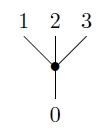Next, we wish to describe how to combine the operads $[0,\infty)$ and ${Com}$ to obtain the phylogenetic operad. Any pair of operads $O$ and $O'$ has a coproduct $O + O'$. The definition of coproduct gives an easy way to understand the algebras of $O + O'$. Such an algebra is simply an object that is both an algebra of $O$ and an algebra of $O'$, with no compatibility conditions imposed.

One can also give an explicit construction of $O + O'$. Briefly, the $n$-ary operations of $O + O'$ are certain equivalence classes of trees with leaves labelled $\{1,\dots, n\}$ and all nodes, except for leaves, labelled by operations in $O$ or $O'$. While this fact is surely known to experts on operads, it seems hard to find in the literature, so we prove this in Theorem 24. Cat lovers out there will be pleased to note that the proof relies on the existence of an algebraic theory whose algebras are operads.

Given this, it should come as no surprise that the operad ${Phyl}$ is the coproduct ${Com} + [0,\infty)$. In fact, we shall take this as a definition. Starting from this definition, we work backwards to show that the operations of ${Phyl}$ correspond to phylogenetic trees. We prove this in Theorem 28. The definition of coproduct determines a topology on the spaces ${Phyl}_n$, and it is a nontrivial fact that with this topology we have ${Phyl}_n \cong \mathcal{T}_n \times [0,\infty)^{n+1}$ for $n \gt 1$, where $\mathcal{T}_n$ has the topology defined by Billera, Holmes and Vogtmann. We prove this in Theorem 34.

Using the definition of the phylogenetic operad as a coproduct, it is clear that given any Markov process on a finite set $X$, the vector space $\mathbb{R}^X$ naturally becomes a coalgebra of this operad. The reason is that, as we have seen, $\mathbb{R}^X$ is automatically a coalgebra of ${Com}$, and the Markov process makes it into a coalgebra of $[0,\infty)$. Thus, by the universal property of a coproduct, it becomes a coalgebra of ${Phyl} \cong {Com} + [0,\infty)$. We prove this in Theorem 36.

Since operads arose in algebraic topology, it is interesting to consider how the phylogenetic operad connects to ideas from that subject. Boardmann and Vogt defined a construction on operads, the ‘$W$ construction’, which when applied to the operad for spaces with an associative multiplication gives an operad for loop spaces. The operad ${Phyl}$ has an interesting relation to $W({Com})$. To see this, define addition on $[0,\infty]$ in the obvious way, where

$\infty + t = t + \infty = \infty$

Then $[0,\infty]$ becomes a commutative topological monoid, so we obtain an operad with only unary operations, one for each $t \in [0,\infty]$, where composition is addition. By abuse of notation, let us call this operad $[0,\infty]$.

Boardmann and Vogt’s $W$ construction involves trees with edges having lengths in $[0,1]$, but we can equivalently use $[0,\infty]$. Leinster has observed that for any nonsymmetric topological operad $O$, Boardmann and Vogt’s operad $W(O)$ is closely related to $O + [0,\infty]$. Here we make this observation precise in the symmetric case. Operations in ${Com} + [0,\infty]$ are just like phylogenetic trees except that edges may have length $\infty$. Moreover, for any operad $O$, the operad $W(O)$ is a non-unital suboperad of $O + [0,\infty]$. An operation of $O + [0,\infty]$ lies in $W(O)$ if and only if all the external edges of the corresponding tree have length $\infty$. We prove this in Theorem 40.

Berger and Moerdijk showed that if $S_n$ acts freely on $O_n$ and $O_1$ is well-pointed, then $W(O)$ is a cofibrant replacement for $O$. This is true for $O = {Assoc}$, the operad whose algebras are topological semigroups. This cofibrancy is why Boardmann and Vogt could use $W({Assoc})$ as an operad for loop spaces. But $S_n$ does not act freely on ${Com}_n$, and $W({Com})$ is not a cofibrant replacement for ${Com}$. So, it is not an operad for infinite loop spaces.

Nonetheless, the larger operad ${Com} + [0,\infty]$, a compactification of ${Phyl} = {Com} + [0,\infty)$, is somewhat interesting. The reason is that any Markov process

$\alpha \colon [0,\infty) \to \mathrm{End}(\mathbb{R}^X)$

approaches a limit as $t \to \infty$. Indeed, $\alpha$ extends uniquely to a homomorphism from the topological monoid $[0,\infty]$ to $\mathrm{End}(\mathbb{R}^X)$. Thus, given a Markov process on a finite set $X$, the vector space $\mathbb{R}^X$ naturally becomes a coalgebra of ${Com} + [0,\infty]$. We prove this in Theorem 38.

Posted at December 22, 2015 9:06 PM UTC

TrackBack URL for this Entry:   https://golem.ph.utexas.edu/cgi-bin/MT-3.0/dxy-tb.fcgi/2857

### Re: Operads and Phylogenetic Trees

This is very cool! It’s neat that “Markov processes with branching” used in genomics have a nice operadic description, and it’s tantalizing that there might be some connection to the original application of operads (iterated loop spaces).

As a step in the latter direction, we might note that if $\tilde{C}$ is a $\Sigma$-cofibrant replacement for $Com$ (that is, $S_n$ acts freely on $\tilde{C}_n$ and we have a weak equivalence $\tilde{C}\to Com$), then any $Com$-(co)algebra becomes automatically an $\tilde{C}$-(co)algebra. Therefore, the phylogenetic Markov models should also yield $(\tilde{C}+[0,\infty])$-coalgebras, while $W(\tilde{C})$ would be an operad for infinite loop spaces. Then if we could apply some natural contravariant monoidal functor from vector spaces to topological spaces, we would get an actual infinite loop space, and we could ask whether it’s anything familiar to algebraic topologists.

Posted by: Mike Shulman on December 26, 2015 3:45 AM | Permalink | Reply to this

### Re: Operads and Phylogenetic Trees

Thanks. That’s an interesting idea. I’m having trouble imagining a $\Sigma$-cofibrant replacement for $Com$ that’s not already an operad for infinite loop spaces. What’s the ‘smallest’, most ‘algebraic’ one that people know? Does one have to bring in the spaces $B\Sigma_n$?

Posted by: John Baez on December 27, 2015 5:27 PM | Permalink | Reply to this

### Re: Operads and Phylogenetic Trees

Fair enough. Probably the $B\Sigma_n$s do give the most “algebraic” example (I think maybe that is called something like the (topological) Barrat-Eccles operad?). But even if $\tilde{C}$ is already an operad for infinite loop spaces, it might be that the presence of the additional $[0,\infty]$ action would yield something interesting at the level of infinite loop spaces.

Posted by: Mike Shulman on December 27, 2015 11:17 PM | Permalink | Reply to this

### Re: Operads and Phylogenetic Trees

I think of cofibrant replacement as a process of ‘puffing up’ an object, replacing equations by paths.

From this viewpoint, replacing an operad $O$ by $O + [0,\infty]$ has an interesting meaning. We are puffing up $O$ by introducing new operations $f_t$ where $t \in [0,\infty]$. If $\mathbb{R}^X$ is an algebra of $O$, we can extend it to an algebra of $O + [0,\infty]$ by putting a Markov process on the set $X$. The operations $f_t$ then describe ‘random walks’ starting at any element of $X$, in a manner that doesn’t respect the structure of $X$ as an $O$-algebra. The operation $f_\infty$ has the effect of ‘completely randomizing’ any element of $X$.

The Boardman–Vogt operad $W(O)$ consists of all operations of $O + [0,\infty]$ corresponding to trees with vertices labelled by operations of $O$, edges labelled by times $t \in [0,\infty]$, and external edges labelled by the time $t = \infty$. These operations are sufficiently randomized that they don’t obey any of the equations one expects from operations in $O$.

Thus, roughly speaking, in $W(O)$ we have puffed up $O$ by replacing equations between operations with ‘random walks’.

But it would be nice to go somewhere with this idea. It’s a nuisance that $W(O)$ is not a cofibrant replacement of $O$ for for my favorite example, $O = \mathrm{Com}$.

Posted by: John Baez on December 28, 2015 1:32 AM | Permalink | Reply to this

### Re: Operads and Phylogenetic Trees

We can take that a step further by recalling the standard sort of algebra-geometry duality. If $X$ is a discrete set, then $\mathbb{R}^X$, with its pointwise $Com$-algebra structure, is the algebra of $\mathbb{R}$-values functions on the “space” $X$. More generally, any $Com$-algebra $A$ can be regarded as the algebra of functions on some space (one such space being the scheme $Spec(A)$ over $Spec(\mathbb{R})$). So if $Com$-algebras are an algebraic representation of a certain kind of space, it might make sense to think of $(Com+[0,\infty])$-algebras as an algebraic representation of “spaces equipped with a Markov process”.

I don’t understand the passage to coalgebras from this point of view, though.

Posted by: Mike Shulman on December 28, 2015 4:19 AM | Permalink | Reply to this

### Re: Operads and Phylogenetic Trees

Since the category of finite-dimensional vector spaces is equivalent to its opposite, there’s no significant difference between a finite-dimensional commutative algebra and a finite-dimensional cocommutative coalgebra.

However, I’m interested in reading a phylogenetic tree as an operation that takes a probability distribution on the genotypes of the original ancestor (the guy at the bottom of the tree) and produces a probability distribution on the genotypes of its descendants today (the guys sitting on the leaves at the top of the tree). At each branch, the duplication

$\begin{array}{ccl} \Delta : & X& \to X \times X \\ &x &\mapsto (x,x) \end{array}$

of the genotype gives rise to a map

$\begin{array}{ccl} &\mathbb{R}^X& \to \mathbb{R}^X \otimes \mathbb{R}^X \\ &f& \mapsto f(-,-) \end{array}$

which lets us ‘duplicate probability distributions’.

So, I’m more interested in the cococommutative coalgebra structure on $\mathbb{R}^X$ than its commutative algebra structure.

Indeed, if in this paper I’d been talking to a narrower audience of nerds I would have written something like $\mathbb{R}[X]$, meaning the free vector space on the set $X$, rather than $\mathbb{R}^X$. Every set is a cocommutative comonoid in a unique way, and this makes the free vector space on that set into a cocommutative coalgebra. Only for a finite set $X$ is this free vector space also the commutative algebra of all $\mathbb{R}$-valued functions on the set, namely $\mathbb{R}^X$.

We could do a fancier version where $X$ was a measure space; then the duplication map would make the space of measures on $X$ into a cocommutative coalgebra.

But I figured that the people who already care about this stuff don’t need to be told, and the people who don’t care yet won’t be converted by reading this particular paper.

Posted by: John Baez on December 29, 2015 12:50 AM | Permalink | Reply to this

### Re: Operads and Phylogenetic Trees

Well, I care about that stuff, but I found the reminder useful. (-: Is there a formal way to regard any cocommutative coalgebra as the space of “measures” on some “measure space”, analogously to how any commutative algebra can be regarded as the space of “functions” on some “space” (such as its Zariski spectrum)?

Posted by: Mike Shulman on January 1, 2016 4:13 AM | Permalink | Reply to this

### Re: Operads and Phylogenetic Trees

Mike wrote:

Is there a formal way to regard any cocommutative coalgebra as the space of “measures” on some “measure space”, analogously to how any commutative algebra can be regarded as the space of “functions” on some “space” (such as its Zariski spectrum)?

Yes. The best of us simply regard cocommutative coalgebras as coalgebras of measures ‘by fiat’, much as Grothendieck decreed that any commutative algebra is the algebra of functions on some ‘affine scheme’… and letting this be the definition of ‘affine scheme’.

Of course, in algebraic geometry there’s a long tradition of imposing various restrictions on commutative algebras to give their corresponding affine schemes various nice properties. I’m not aware of analogous work in ‘coalgebraic geometry’. But someone must have tried!

Posted by: John Baez on January 1, 2016 5:22 PM | Permalink | Reply to this

### Re: Operads and Phylogenetic Trees

Even more convincing to me would be showing that you can do some “measure theory” with arbitrary cocommutative coalgebras.

Posted by: Mike Shulman on January 2, 2016 3:49 AM | Permalink | Reply to this

### Re: Operads and Phylogenetic Trees

The most basic feature of measure theory is integration; we can integrate a finite measure and get a number. For a cocommutative coalgebra, this sort of integration is just the counit.

Another basic feature of measure theory is that it gives a covariant symmetric monoidal functor from measure spaces to cocommutative coalgebras. Defining measure spaces to be cocommutative coalgebras does that quite nicely.The more nuanced features of measure theory tend to involve countable additivity. I don’t think cocommutative coalgebras know about that. So we should probably think of them as formalizing charges rather than measures.

Posted by: John Baez on January 2, 2016 4:16 AM | Permalink | Reply to this

### Re: Operads and Phylogenetic Trees

If I may ask a really dumb question… what is the comultiplication on a space of measures?

Posted by: Mike Shulman on January 2, 2016 10:21 PM | Permalink | Reply to this

### Re: Operads and Phylogenetic Trees

It’s not a dumb question: you’d need to be fairly interested in algebra to ask it, and fairly interested in analysis to know the answer.

In fact, I just realized I’m not quite interested in analysis enough anymore to know the answer.

The basic idea is that if we have a measure on $X$ we can ‘comultiply’ it and get a measure supported on the diagonal of $X \times X$, since this diagonal looks just like $X$.

If we let $M(X)$ is the space of measures on $X$, this comultiplication gives a linear map

$\Delta : M(X) \to M(X \times X)$

We’d be done if

$M(X) \otimes M(X) \cong M(X \times X)$

But this isn’t true with the usual ‘algebraic’ tensor product of vector spaces, unless $X$ is a finite set. In general $M(X \times X)$ is bigger than $M(X) \otimes M(X)$. So, we need to ‘complete’ the algebraic tensor product $M(X) \otimes M(X)$ in some careful way to get $M(X \otimes X)$.

There are probably a bunch of different contexts in which one can make this stuff precise, but let’s work with compact Hausdorff spaces. Given such a space $X$, there’s a commutative unital C*-algebra of continuous real-valued functions on $X$, called $C(X)$. In particular this is a Banach space. The space of continuous linear functionals on this Banach space is called $C(X)^*$. But it’s also called $M(X)$, since by the Riesz–Markov theorem it’s naturally isomorphic to the space of finite Borel measures on $X$.

The point is that any such measure lets you integrate a continuous function on $X$ and get a number. So, you can check it gives an element of $C(X)^*$. And conversely, any element of $C(X)^*$ gives a finite Borel measure: that’s the hard part.

Thinking of these measures as linear functionals makes it easy to describe the comultiplication of measures as a map

$\Delta : M(X) \to M(X \times X)$

Namely, if $\mu \in M(X)$, $\Delta(\mu)$ is the continuous linear functional that sends $f \in C(X \times X)$ to

$\int_X f(x,x) d\mu (x)$

So, if you want to get involved with it, the remaining work is to figure out the correct ‘completed tensor product’ for spaces of measures that lets us say

$M(X \times Y) \cong M(X) \hat{\otimes} M(Y)$

and turn our comultiplication into a map

$\Delta : M(X) \to M(X) \hat{\otimes} M(X)$

I forget how this goes. In fact I’m not sure I ever knew! I used to know the answer for the dual problem, the tensor product of C*-algebras that lets you say

$C(X \times Y) \cong C(X) \hat{\otimes} C(Y)$

I believe it’s discussed in Takesaki’s book on operator algebras. But anyway, for some purposes you can circumvent this crud by cheating and simply defining the completion of $M(X) \otimes M(Y)$ to be $M(X \times Y)$.

So you see, that wasn’t a dumb question.

At least you didn’t say “I have a quick question.” I hate it when people say that. It makes me wanna say “Sure, buddy, the question is quick, but you left me stuck with answering it.”

Posted by: John Baez on January 2, 2016 11:40 PM | Permalink | Reply to this

### Re: Operads and Phylogenetic Trees

A hit-and-run comment before crashing into bed:

Coalgebras of Banach-ish things are problematic because the usual tensor product (the canonical one for the category of Banach spaces) is often too small. But in this particular case, I think one can take the tensor product in what Cigler-Losert-Michor called the category of “Waelbroeck spaces” (this is, IIRC, equivalent to the opposite of the category of Banach spaces). A more pedestrian way to think of this category and its tensor product is that the objects should be dual Banach spaces where we remember the weak-star topology on the unit ball and morphisms are the bounded weak-star continuous maps; and then I think that Cigler-Losert-Michor observe somewhere in their book that this category has a symmetric monoidal structure, with the tensor being such that M(X) tensor M(Y) is M(X times Y).

An aside: this tensor product linearizes the bilinear maps which are jointly weakstar-to-weakstar continuous in both variables; in various settings in functional analysis, we’d like to have something similar which can linearize the bilinear maps which are separately weakstar-to-weakstar continuous in each variable, but AFAIK efforts in this direction lead to a World of Pain…

(The tensor of the preduals which you refer to is the injective tensor product of Banach spaces, which for C(K) spaces coincides with the minimal tensor product of Cstar algebras.)

Posted by: Yemon Choi on January 3, 2016 5:09 AM | Permalink | Reply to this

### Re: Operads and Phylogenetic Trees

There’s got to be a fairly nice way to make it full and also faithful. I’m a bit too lazy to work out the details.

Posted by: John Baez on January 3, 2016 10:02 PM | Permalink | Reply to this

### Re: Operads and Phylogenetic Trees

Ah, ok; thanks! So basically we choose the codomain of the functor $M$ (including its tensor product) so that $M$ becomes strong monoidal, hence preserves cocommutative comonoids, then use the fact that everything in a cartesian monoidal category is a cocommutative comonoid in a unique way.

Is this resulting functor $M$ a full embedding of some category of measurable spaces into the appropriate category of comonoids?

Posted by: Mike Shulman on January 3, 2016 9:57 PM | Permalink | Reply to this

### Re: Operads and Phylogenetic Trees

Jack Morava pointed out these related papers:

Abstract: The orientable cover of the moduli space of real genus zero algebraic curves with marked points is a compact aspherical manifold tiled by associahedra, which resolves the singularities of the space of phylogenetic trees. The resolution maps planar metric trees to their underlying abstract representatives, collapsing and folding an explicit geometric decomposition of the moduli space into cubes. This decomposition endows the resolving space with an interesting canonical pseudometric. The second part of this paper defines a related (stacky) resolution of a space of real quadratic forms, and suggests, perhaps without much justification, that systems of oscillators parametrized by such objects may provide useful models in genomics.

Abstract. In a previous paper, we showed that the orientable cover of the moduli space of real genus zero algebraic curves with marked points is a compact aspherical manifold tiled by associahedra, which resolves the singularities of the space of phylogenetic trees. In this draft of a sequel, we construct a related (stacky) resolution of a space of real quadratic forms, and suggest, perhaps without much justification, that systems of oscillators parametrized by such objects may may provide useful models in genomics.

Posted by: John Baez on December 28, 2015 1:47 AM | Permalink | Reply to this

### Re: Operads and Phylogenetic Trees

This notion of “coalgebra” for an operad is a bit unexpected, at least for me, in that if an operad has only unary operations (i.e. is just an $\mathbb{R}$-algebra $A$) then its coalgebras are the same as its algebras (both coinciding with the $A$-modules, and neither one coinciding with the $A$-comodules). Is this the standard meaning of “coalgebra for an operad”? Is the version that would specialize to comodules ever studied?

Posted by: Mike Shulman on December 28, 2015 4:12 AM | Permalink | Reply to this

### Re: Operads and Phylogenetic Trees

To ask what I know is a semi-heretical question: are there any immediate applications back to biology of thinking of phylogenetic trees operadically (not that the math isn’t nice on its own!)?

Posted by: Greg Friedman on December 28, 2015 8:34 AM | Permalink | Reply to this

### Re: Operads and Phylogenetic Trees

I think that an immediate application would be to extend this operadic way of thinking to find solutions to other ‘parameterization problems’ in phylogenetics or biology. For example, to parametrize phylogenetic trees that have both labelled and dead leaves (to take into account also extinction), we just have to use the terminal operad instead of the operad for commutative semigroups. For another example, by using PROPs instead of operads, we could get a space that parametrizes phylogenetic networks.

Posted by: Nina Otter on December 28, 2015 1:38 PM | Permalink | Reply to this

### Re: Operads and Phylogenetic Trees

Could another possible extension be to the set of sampled ancestor trees (http://journals.plos.org/ploscompbiol/article?id=10.1371/journal.pcbi.1003919), where taxa are allowed to be internal nodes as well as degree-two nodes? That would be cool I think!

Posted by: Alex Gavryushkin on December 28, 2015 7:58 PM | Permalink | Reply to this

### Re: Operads and Phylogenetic Trees

That’s a neat result!

Ultrametric trees are different indeed. There are several ways they can be modelled along the lines of the BHV approach. Different ways result in different polytopal complexes:
http://arxiv.org/abs/1410.3544
Some are more natural than others:
http://arxiv.org/abs/1510.08797

I’m wondering if Phyl can naturally be ‘restricted’ to ultrametric trees?

Posted by: Alex Gavryushkin on December 28, 2015 7:37 PM | Permalink | Reply to this

### Re: Operads and Phylogenetic Trees

Are rooted ultrametric trees with $n$ labelled leaves the $n$-ary operations of some operad?

That is, can we graft the root of such a tree with $n$ labelled leaves onto a leaf of another such tree with $m$ labelled leaves and get a rooted ultrametric tree with $m+n-1$ labelled leaves?

I seem to recall thinking the answer was no. But I also seem to recall thinking the answer was yes and that this corresponds to some known operation on ultrametric spaces. Right now I’m feeling too lazy to figure it out.

Posted by: John Baez on December 29, 2015 1:02 AM | Permalink | Reply to this

### Re: Operads and Phylogenetic Trees

Joachim Kock sent me this email, which he has allowed me to reprint here. I hope someone takes advantage of these ideas to improve the treatment of trees in operad theory!

Hi John,

Happy new year!

In Torino we had the opportunity to discuss directed graphs.
with Nina Otter, I send you some similar remarks for trees.
I do realise that this kind of email classifies as spam :-(
For this reason I do not Cc Nina (whom I never met).  Feel free
to share with her, if there is anything that could be of
interest.  Most of what I write is probably too trivial, but
that is the level I like the most.

I feel a bit like a religious fanatic trying to convert innocent
people.  My strategy is to make them answer yes to some easy
questions and then impose the consequences upon them :-)

(1) Grafting (gluing) should be expressed as colimits in a
category.  (Yes.)

(2) Subtrees should be given by inclusions which should be
morphisms in that category.  (Yes.)

(3) The category should contain the trivial tree, consisting of
a single edge and no nodes.  (Yes.)

These three points are closely related: grafting should be a
pushout of the inclusion of the trivial tree into the bottom
tree as a leaf and the inclusion of the trivial tree into the
top tree as the root.

(4) A tree should be implemented so as to have certain features:
leaves, root, set of incoming edges of each node (arity),
outgoing edge of each node, notion of inner edges, and of course
the incidences.

Having all this data would be redundant, as some parts of the
data induce other parts.

We want to present the data in such a way so as to get a natural
notion of morphism, as needed in (1).  The kind of subtree
inclusions we want should send edges to edges and nodes to
nodes, and be arity preserving.  Obviously it should not
be required to preserve leaves or root.

We have to encode the tree data in such a way that root and
leaves are implicit, not explicit.  Here is my solution, which I
take every opportunity to advertise :-)

Everything here is from my paper "Polynomial functors and trees",
IMRN 2011, arXiv:0807.2874.

A tree is a diagram

s     p     t
A <-- M --> N --> A

subject to 3 axioms.  A is the set of edges, N is the set of
nodes, M is the set of nodes with a marked incoming edge,
so that the set of incoming edges of a given node x is the
fibre M_x.  t returns the outgoing edge of a node, and s returns
the marked edge.  p forgets the mark.

The axioms are

1: t is injective (meaning that an edge is outgoing of at
most one node.  (The complement of t is the set of leaves.)

2: s is injective with singleton complement.  This complement
is the root, and is denoted 1.

3.  With A=M+1, consider the walk-towards-the-root function
sigma : A -> A which sends 1 to 1, and sends x in M to tpx.
The axiom now say that for every x in A there is a natural
number k such that sigma^k x = 1.

Definition: a morphism of trees is a diagram

A'<-- M'--> N'--> A'
|     |     |     |     (*)
v     v     v     v
A <-- M --> N --> A

in which the middle square is a pullback.  (This expresses
arity preservation).

One can show that such a morphism is automatically levelwise
injective. This follows from the axioms.  These morphisms
are the required tree inclusions.

I am very proud of this.

A trivial tree is

1 <-- 0 --> 0 --> 1

The inclusion of the trivial tree into another tree is
a leaf precisely when also the right-hand square is a
pullback, and it is a root when instead also the left-hand
square is a pullback.  More generally, morphisms in which
the right-hand square is a pullback are leaf-preserving,
and morphisms in which the left-hand square is a pullback
are root preserving.

Lemma.  Leaf-preserving and root-preserving maps admit
pushout along each other, and the result is again leaf-
preserving resp. root-preserving.

Grafting is now a pushout.

Comparison with Baez-Otter trees:

[BO] notion V is my N.

[BO] notion E is my A.

[BO] notion {0} is not represented as a vertex anywhere in
my formalism, but the map t: E -> V+{0} is: the inverse
image t^{-1}(0) is my 1; removing it from E=A gives my M
with natural inclusion into A (remembering that A=M+1)
which is my s.

The [BO] map t restricts to my p: M -> N.

The [BO] bijection s: E -> V+{1,2,...,n} can be inverted,
and the restriction to V is my t: N -> A.  Under the [BO]
bijection s, {1,2,...,n} can be identified with a subset of the
edge set, so is not needed.  It is implicit as the complement
of the mono s.

Under the singletonness of t^{-1}(0), the [BO] 0 is identified
with an element in E (my 1 in A), so is not needed either.

The set of inner edges of a tree in my sense is the pullback
N \times_A M (really an intersection of two monos).

Now there is of course the question of the numbers labeling the
leaves.  Their role is not motivated by any natural numbering of
the present-day species.  It is only needed to comply with the
convention that operads have their inputs numbered.  That's a
convention in turn motivated only by our habit of writing things
linearly.  It is not actually an essential aspect of operads.
An elegant way of saying what an operad is is to say: for every
finite set I a space O_I of I-indexed operations, and for every
surjection I ->> J a composition map O_I -> O_J, etc.
You know this, of course.  One reference is Spivak.

But assuming that the numbers are needed, let us simply decorate
the leaves manually, on top of the basic structure.  This is ad
hoc, but it seems to me that the later the better for ad hoc
additions.  For many constructions the numberings are not
needed.

For example, for most of the combinatorics, you actually deal
with planar trees.  For planar trees, the numbering on the
leaves is implied, and it seems to me that it is better left as
something implied, rather than carrying it around in the
constructions.  It is cumbersome to re-number the leaves when
grafting or when taking a subtree.  In constrast the planar
structure takes care of itself, and in a rather elegant manner
with the above formalism.  But to explain this, a short
digression is needed.

The really cool feature of the tree formalism is the fact that
the shape of a tree diagram is precisely the shape of a
polynomial endofunctor!  A polynomial is a diagram

s     p     t
I <-- E --> B --> I

without conditions.  It defines the polynomial endofunctor

t_! p_* s^* : Set/I ---> Set/I.

The one-variable case I=1 is already very interesting.  The
finitary ones are precisely the sigma-cofibrant analytic
functors, so finitary polynomial monads are the sigma-cofibrant
steps up one categorical dimension, from sets to groupoids,
everything is sigma-cofibrant, and (finitary) polynomial
functors are the same thing as groupoid-analytic functors, in
turn the same thing as stuff types...

For P a polynomial functor, a P-tree is by definition a tree
with a cartesian map to P (meaning that the middle square in (*)
is a pullback).  P-trees can also be characterised as the set of
operations for the free monad on P, or as the initial
(1+P)-algebra.  These have recursive characterisations; it is
sort of surprising (and very useful) that the above tree
formalism provides this direct combinatorial characterisation of
P-trees.

Let P be the free-monoid monad, which is polynomial, represented
by the diagram

1 <-- N'--> N --> 1

where N is the set of natural numbers and N' is the set of
pointed natural numbers, so that the fibre over n is an ordered
n-element set.

Then P-trees are precisely planar trees.

The characterisations of leaves, root, inclusions, pushouts
and hence grafting are the same for P-trees as for naked trees.

(By the way, there is a slight annoyance, that over Set, naked
trees are not P-trees for any P.  Such a P should be the terminal
polynomial endofunctor (under cartesian natural transformations)
but this terminal object does not exists.  It does exists (at
least for finitary polynomial functors) if we upgrade to
groupoids!), then it is the exponential functor, corresponding
to the terminal stuff type.)

Sorry for being long!

Cheers,
Joachim.

Posted by: John Baez on January 2, 2016 1:38 AM | Permalink | Reply to this

Post a New Comment